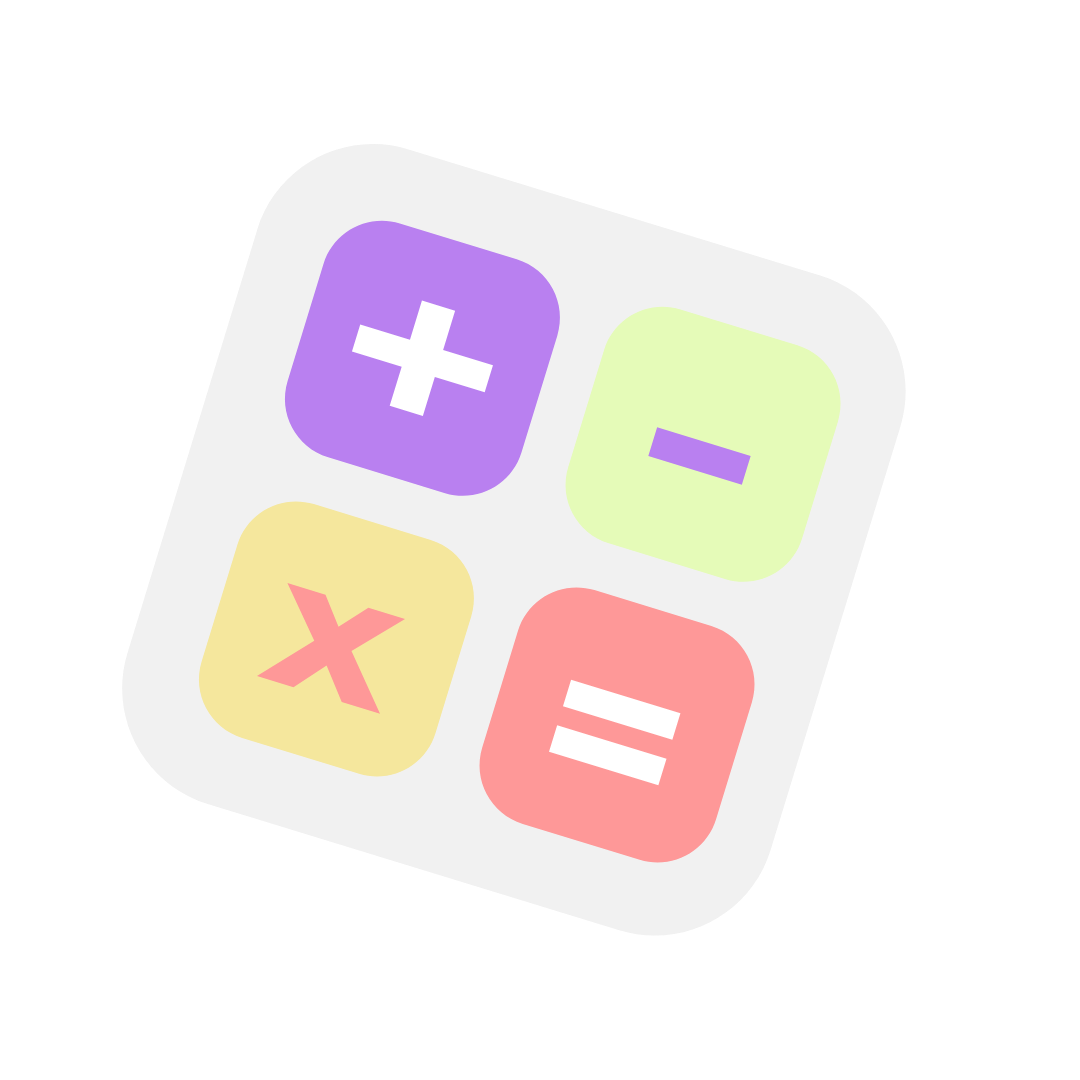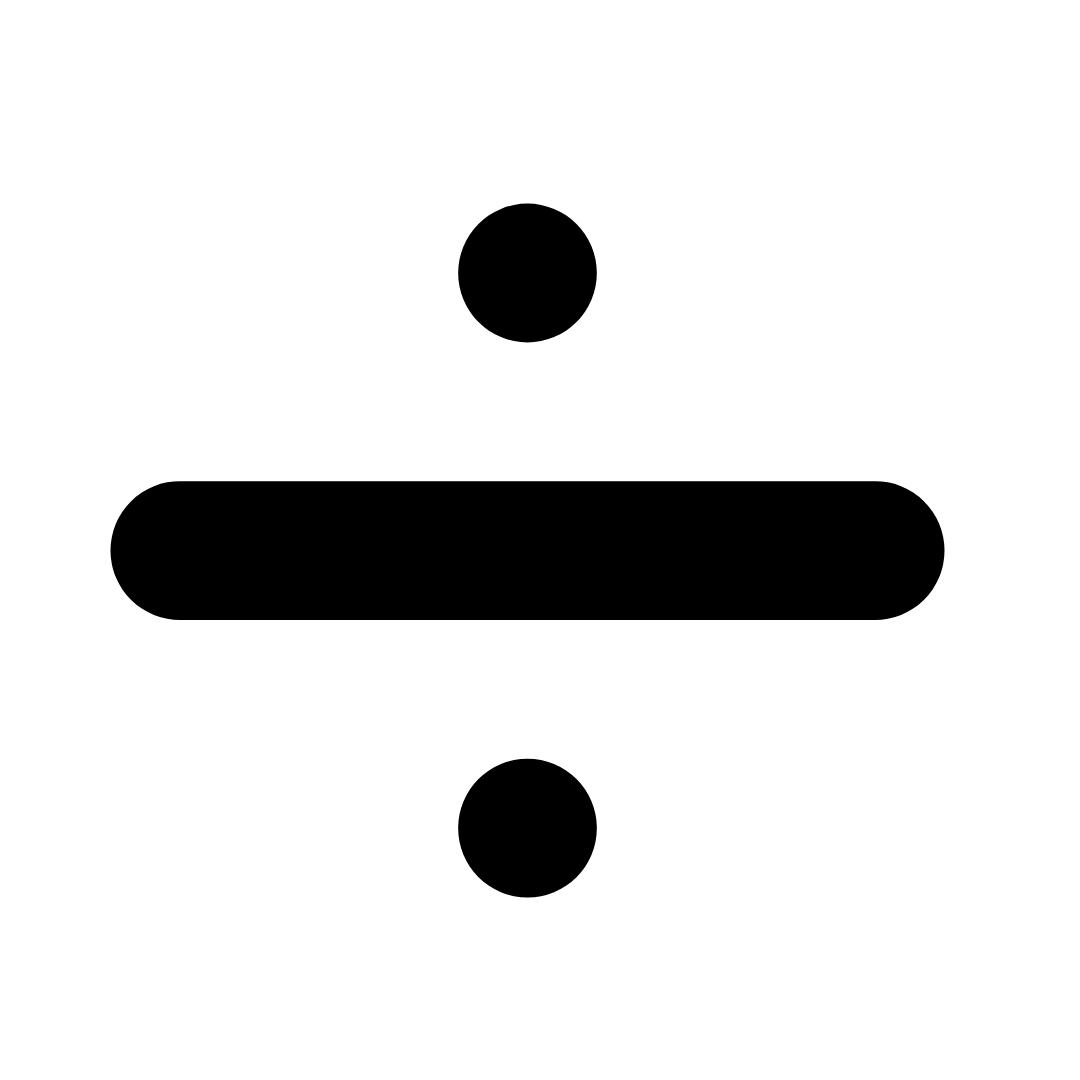Courses
Courses for Kids
Free study material
Free LIVE classes
More

# Maths Worksheet for Kids- Algebra Practice ProblemsLIVE
Join Vedantu’s FREE Mastercalss

## Maths Worksheet for Kids| Algebra Practice Problems - Download Free PDF with Solutions

Teaching the students of KG-3 about algebra is the best way to introduce them to a new topic. Algebra is a crucial topic that not only forms the syllabus of KG-3 Maths but also assists them in higher studies. Solving the Algebra practice problems will enable students to learn the topic easily. Students will get good foundational knowledge about the topics and concepts of algebra in KG-3. This will enable them to solve the questions easily. Students can download the worksheets for Algebra and solve the Algebra Practice Problems to solve the equations properly.

Algebra word problems are pretty common amongst KG-3 students in which they will learn to form different equations using the Algebraic variables. This is a very important branch of mathematics that will help students deal with the rules for manipulating the variables in the best way. Students can combine the concepts and solve the questions easily and properly with the worksheets for Algebra.

Last updated date: 03rd Oct 2023
Total views: 21.6k
Views today: 1.21k

## Access Worksheet for Maths KG-2 Algebra Practice Problems

The basic ways of presenting the unknown values as variables help to create mathematical expressions. Basics of Algebra cover the simple operation of mathematics like addition, subtraction, multiplication, and division.

Solve questions for understanding -operations of Mathematics

### Questions:

15 + 4 = ?

23 +12 = ?

2. Divide.

24 ÷ 6 = ?

15 ÷ 3 = ?

3. Subtract.

25 - 5 = ?

64 - 34 = ?

4. Multiply.

8 × 3 = ?

10 × 5 = ?

5. Solve.

2 × 8 - 5 = ?

35 ÷ 7 + 49 = ?

6. Divide.division symbol

40 ÷ 4 = ?

66 ÷ 3 = ?

7. Subtract.subtraction with symbol

26 - 5 = ?

38 - 23 = ?

8. Multiply.

21 × 2 = ?

17 × 6 = ?

47 + 14 = ?

35 + 25 = ?

10. Solve.

56 + 89 - 37 = ?

54 × 4 - 70 = ?

11. Subtract.

83 - 40 = ?

17 - 8 = ?

12. Divide.

14 ÷ 7 = ?

36 ÷ 2 = ?67 + 34 = ?

38 + 32 = ?

14. Multiply.multiplication symbol

24 × 2 = ?

15 × 3 = ?

15. Solve.

60 ÷ 3 - 9 = ?

45 - 39 + 16 = ?

1.

19, If we add 4 in 15 then we get 19.

35, If we add 12 in 23 then we get 35.

2.

4, If we divide 24 by 6 then we get 4.

5, If we divide 15 by 3 then we get 5.

3.

20, If we subtract 5 from 25 then we get 20.

30, If we subtract 34 from 64 then we get 30.

4.

24, if we multiply 8 with 3 then we get 24.

50, If we multiply 10 with 5 then we get 50.

5.

11, If we multiply 2 with 8 then subtract 5 from it then we get 11.

54, If we divide 35 by 7 then add 49 in it then we get 54.

6.

10, If we divide 40 by 4 then we get 10.

22, If we divide 66 by 3 then we get 22.

7.

21, If we subtract 5 from 26 then we get 21.

15, If we subtract 23 from 38 then we get 15.

8.

42, If we multiply 21 with 2 then we get 42.

102, If we multiply 17 with 6 then we get 102.

9.

61, If we add 14 in 47 then we get 61.

60, If we add 25 in 35 then we get 60.

10.

108, If we add 89 in 56 then subtract 37 from it then we get 108.

146, If we multiply 54 with 4 then subtract 70 from it then we get 146.

11.

43, If we subtract 40 from 83 then we get 43.

9, If we subtract 8 from 17 then we get 9.

12.

2, If we divide 14 by 7 then we get 2.

18, If we divide 36 by 2 then we get 18.

13.

101, If we add 34 in 67 then we get 101.

70, If we add 38 in 32 then we get 70.

14.

48, If we multiply 24 with 2 then we get 48.

45, If we multiply 15 with 3 then we get 45.

15.

11, If we divide 60 by 3 then subtract 9 from it then we get 11.

22, If we subtract 39 from 45 then add 16 in it then we get 22.

### Significance of Algebra Worksheets for Students

• Algebra is something that we see in our daily lives, and that too without realising it. Solving the questions from Algebra worksheets will help the students relate and understand the proper relevance of algebra in real life.

• Algebra can help people in different aspects, such as measuring, finance, and other important things. That is why this topic is considered the most important for students.

• Teaching KG-3 students about the concepts of Algebra will enable them to retain the information in the best way. They can practice the worksheets and prepare for higher studies as well.

• These worksheets will also help increase the logical thinking of students as well. They can break down the problems easily and find the proper solutions to complete the topic.

### Benefits of Algebra Questions and Answers PDF

• To ensure students can have the best learning experience, the experts at Vedantu have created the Algebra Addition and Subtraction Worksheets PDF. Students will get a clear overview of the topic from these worksheets.

• The worksheet has different activities and exercises to make learning fun and interesting for the students. The formulation of these worksheets has been done with extreme care to ensure the comfort of students.

• By solving the questions from Algebra Questions and Answers PDF, students can gain insights into forming different equations on their own with the help of Algebraic variables in the best way.

• Students can download the PDF files of worksheets from the official website of Vedantu for free. They can download the worksheets and access them offline. This means they can easily practice the sums whenever they want.

## Learn About Algebra With Worksheets from Vedantu

The professionals from Vedantu have designed the Algebra Questions and Answers PDF for the students of Class KG-3. This is the perfect time to download and practice the worksheets and gain more knowledge about Algebra. Students will be able to solve the word problems by deciphering the meaning and using the algebraic variables properly. Download the worksheets and solutions from Vedantu and prepare the topic well.

## FAQs on Maths Worksheet for Kids- Algebra Practice Problems

1. There are T number of pens in a single pack. If I buy four different packs, how many pens will I have?

Based on the concepts of Algebra, the total number of pens I will have will be 4T.

2. How can I prepare for exams with Algebra Practice Problems?